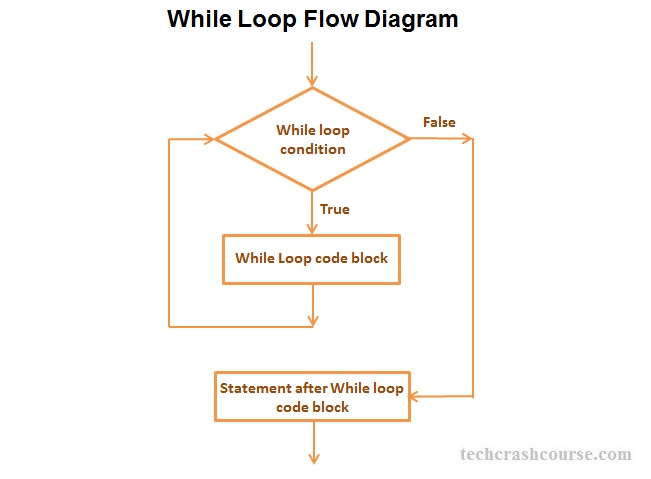# C++ While Loop

The while loop in C++ used for repetitive execution of the statements until the given condition is true. Unlike for loop in C++, there is no initialization and update statements in while loop.

## Syntax of while Loop in C++

while(condition) {
// statements to be executed
}

While Loop Syntax Description
• condition : Condition expression is evaluated before code block of while loop. It is a boolean expression which decides whether to go inside the loop or terminate while loop. If condition expression evaluates to true, the code block inside while loop gets executed otherwise it will terminate while loop and control goes to the next statement after the while loop.
• statement : While loop can contain any number of statements including zero statements. while loop body should change the values checked in the condition in some way, so as to force the condition to become false at some point. Otherwise, the loop will never terminate.

## Flow Diagram of while Loop StatementWhile loop evaluate the condition expression. If the value of condition expression is true then code block of while loop(statements inside {} braces) will be executed otherwise the loop will be terminated. While loop iteration continues unless condition expression becomes false or while look gets terminated using break statement.

## C++ While Loop Example Program

```#include <iostream>
using namespace std;

int main(){

int N, i, sum=0;
cout << "Enter a positive number\n";
cin >> N;
/*
* Initializing loop control variable before while loop
*/
i = 1;
while(i <= N){
// Below statements will only execute if while loop
// condition evaluates to true
sum+= i;
//Incrementing loop control variable
i++;
}
cout << "Sum of Integers from 1 to %d = %d", N, sum;

return 0;
}
```

Output
```Enter a positive number
5
Sum of Integers from 1 to 5 = 15
```
```Enter a positive number
7
Sum of Integers from 1 to 7 = 28
```
In above program, we first take an integer N as input from user. We want to find sum of all integer from 1 to N. Here, we are using while loop to traverse all elements from i=1 to N. While loop will terminate when i becomes > N.
While Loop Interesting Facts
• The condition_expression in while loop is a boolean expression. It must evaluate to true or false value(In C++, all non-zero values are considered as true and zero is considered as false).

• Unlike for loop, initialization statements, condition expression and update statements are on different line.

• Unlike do..while loop, the body of while might not executes even once.
• Opening and Closing braces are not required for single statement inside while loop code block.
For Example:
while(i < 100)
sum+= i;

• We can also use infinite while loops like while(1), which will never terminate. You should add terminating condition using break statement in order to terminate infinite while loop.
For Example:
while(1) {
if(.....){
break;
}
}